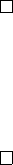8Matrix groups

IA Groups8.1 General and special linear groups
Consider
M
n×n
(
F
), i.e. the set of
n × n
matrices over the field
F
=
R
or
C
(or
F
p
). We know that matrix multiplication is associative (since they represent
functions) but are, in general, not commutative. To make this a group, we want
the identity matrix
I
to be the identity. To ensure everything has an inverse, we
can only include invertible matrices.
(We do not necessarily need to take
I
as the identity of the group. We can,
for example, take
e
=
0 0
0 1
and obtain a group in which every matrix is of
the form
0 0
0 a
for some non-zero
a
. This forms a group, albeit a boring one
(it is simply
=
R
))
Definition (General linear group GL
n
(F )).
GL
n
(F ) = {A M
n×n
(F ) : A is invertible}
is the general linear group.
Alternatively, we can define
GL
n
(
F
) as matrices with non-zero determinants.
Proposition. GL
n
(F ) is a group.
Proof.
Identity is
I
, which is in
GL
n
(
F
) by definition (
I
is its self-inverse). The
composition of invertible matrices is invertible, so is closed. Inverse exist by
definition. Multiplication is associative.
Proposition. det : GL
n
(F ) F \ {0} is a surjective group homomorphism.
Proof. det AB
=
det A det B
. If
A
is invertible, it has non-zero determinant and
det A F \ {0}.
To show it is surjective, for any
x F \ {
0
}
, if we take the identity matrix
and replace I
11
with x, then the determinant is x. So it is surjective.
Definition
(Special linear group
SL
n
(
F
))
.
The special linear group
SL
n
(
F
) is
the kernel of the determinant, i.e.
SL
n
(F ) = {A GL
n
(F ) : det A = 1}.
So SL
n
(F ) C GL
n
(F ) as it is a kernel. Note that Q
8
SL
2
(C)